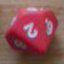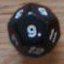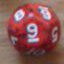Exploding dice and probability of max 1x instance of any n

## Exploding dice and probability of max 1x instance of any n

 Sat Apr 25 14:25:43 2020   by   Ben How can I figure out the probabilities of scoring maximum one occurence of any number in exploding dice?Eg. exploding 2D4 rolling a not more than 1x of: "1" or "2" versus exploding 3d4 rolling max. 2x of "1" Sun Apr 26 14:42:32 2020   by   Torben I assume that you explode on a 4.  The set of dice value you get from exploding N d4 is written as`N#(accumulate x:=d4 while x=4)`To count the number of 1s and 2s in this, you write`count 3 > N#(accumulate x:=d4 while x=4)`But it is fairly easy to see that this is the same as counting the number of 1s and 2s in a non-exploding d3:`count 3 > N d3`The reason is that N exploding d4s have any number of 4s and exactly N values from 1 to 3, with equal probability.